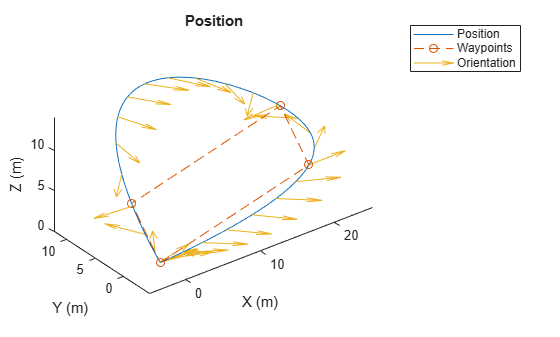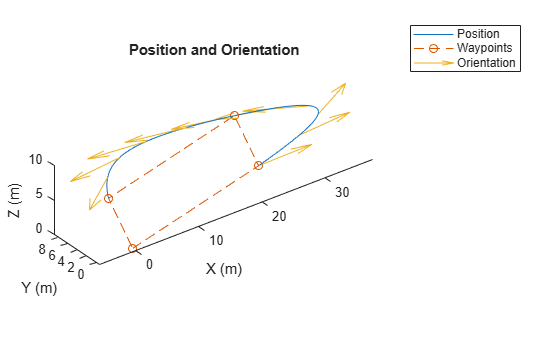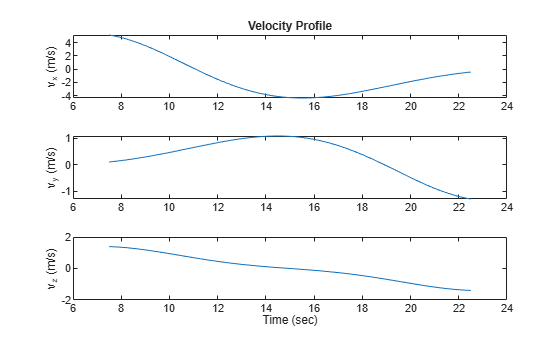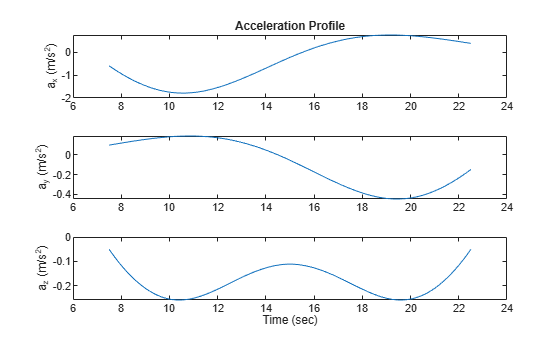# polynomialTrajectory

Piecewise-polynomial trajectory generator

Since R2023a

## Description

The `polynomialTrajectory` System object™ generates trajectories using a specified piecewise polynomial.

You can create a piecewise-polynomial structure using trajectory generators like `minjerkpolytraj`, `minsnappolytraj`, and `cubicpolytraj`, as well as any custom trajectory generator. You can then pass the structure to the `polynomialTrajectory` System object to create a trajectory interface for scenario simulation using the `robotScenario` object.

To generate a trajectory from a piecewise polynomial:

1. Create the `polynomialTrajectory` object and set its properties.

2. Call the object with arguments, as if it were a function.

## Creation

### Syntax

``trajectory = polynomialTrajectory(pp,Name=Value)``

### Description

example

````trajectory = polynomialTrajectory(pp,Name=Value)` returns a System object, `trajectory`, that generates a trajectory using the piecewise polynomial `pp`. Specify properties using one or more name-value arguments. Properties that you do not specify retain their default values.```

### Input Arguments

expand all

Piecewise polynomial, specified as a structure that defines the polynomial for each section of the piecewise trajectory.

Data Types: `struct`

## Properties

expand all

Unless otherwise indicated, properties are nontunable, which means you cannot change their values after calling the object. Objects lock when you call them, and the `release` function unlocks them.

If a property is tunable, you can change its value at any time.

Sample rate of the trajectory in Hz, specified as a positive scalar.

Tunable: Yes

Data Types: `double`

Number of samples per output frame, specified as a positive integer.

Data Types: `double`

Orientation at each waypoint, specified as an N-element `quaternion` column vector or 3-by-3-by-N array of real numbers. N is the number of waypoints.

Each `quaternion` must have a norm of `1`. Each 3-by-3 rotation matrix must be an orthonormal matrix. Each quaternion or rotation matrix is a frame rotation from the local navigation coordinate system to the current body coordinate system at the corresponding waypoint.

If you do not specify this property, then the object sets yaw to the direction of travel at each waypoint, and pitch and roll are subject to the values of the AutoPitch and AutoBank properties, respectively.

Data Types: `double`

Positions in the navigation coordinate system, in meters, specified as an N-by-3 matrix. The columns of the matrix correspond to the first, second, and third axes, respectively. The rows of the matrix, N, correspond to individual waypoints.

The object infers this value from the piecewise polynomial `pp`.

Data Types: `double`

Timestamp at each waypoint, in seconds, specified as an N-element column vector. The number of samples, N, is the same as the number of samples (rows) in Waypoints property. The value of each element of the vector must be greater than the value of the previous element.

The object infers this value from the piecewise polynomial `pp`.

Data Types: `double`

Align the pitch angle with the direction of motion, specified as a logical `0` (`false`) or `1` (`true`). When specified as `true`, the pitch angle automatically aligns with the direction of motion. If specified as `false`, the object sets the pitch angle to `0` (level orientation).

#### Dependencies

To set this property, you must not specify the Orientation property.

Data Types: `logical`

Align the roll angle to counteract the centripetal force, specified as a logical `0` (`false`) or `1` (`true`). When specified as `true`, the roll angle automatically counteracts the centripetal force. If specified as `false`, the object sets the roll angle to `0` (flat orientation).

#### Dependencies

To set this property, you must not specify the Orientation property.

Data Types: `logical`

Reference frame of the trajectory, specified as `"NED"` (North-East-Down) or `"ENU"` (East-North-Up).

Data Types: `char` | `string`

Velocity in the navigation coordinate system at each waypoint, in meters per second, specified as an N-by-3 matrix. The columns of the matrix correspond to the first, second, and third axes, respectively. The number of samples, N, is the same as the number of samples (rows) in Waypoints property.

The object infers this value from the derivative of the piecewise polynomial `pp`.

Data Types: `double`

Horizontal direction of travel, in degrees, specified as an N-element real vector. The number of samples, N, is the same as the number of samples (rows) in Waypoints property.

The object infers this value from the derivative of the piecewise polynomial `pp`.

Data Types: `double`

Groundspeed at each waypoint, in meters per second, specified as an N-element real vector. The number of samples, N, is the same as the number of samples (rows) in Waypoints property.

The object infers this value from the derivative of the piecewise polynomial `pp`.

Data Types: `double`

Climb Rate at each waypoint, in meters per second, specified as an N-element real vector. The number of samples, N, is the same as the number of samples (rows) in Waypoints property.

The object infers this value from the derivative of the piecewise polynomial `pp`.

Data Types: `double`

## Usage

### Syntax

``[position,orientation,velocity,acceleration,angularVelocity] = trajectory()``

### Description

example

````[position,orientation,velocity,acceleration,angularVelocity] = trajectory()` outputs a frame of trajectory data based on specified creation arguments and properties. The trajectory returns `NaN` for positions and orientations outside the range of the time of arrival.```

### Output Arguments

expand all

Position in the local navigation coordinate system, returned as an M-by-3 matrix in meters.

M is specified by the SamplesPerFrame property.

Data Types: `double`

Orientation in the local navigation coordinate system, returned as an M-element `quaternion` column vector or a 3-by-3-by-M real array.

Each quaternion or 3-by-3 rotation matrix is a frame rotation from the local navigation coordinate system to the current body coordinate system at the corresponding sample.

M is specified by the SamplesPerFrame property.

Data Types: `double`

Velocity in the local navigation coordinate system, returned as an M-by-3 matrix, in meters per second.

M is specified by the SamplesPerFrame property.

Data Types: `double`

Acceleration in the local navigation coordinate system, returned as an M-by-3 matrix, in meters per second squared.

M is specified by the SamplesPerFrame property.

Data Types: `double`

Angular velocity in the local navigation coordinate system, returned as an M-by-3 matrix, in radians per second.

M is specified by the SamplesPerFrame property.

Data Types: `double`

## Object Functions

To use an object function, specify the System object as the first input argument. For example, to release system resources of a System object named `obj`, use this syntax:

`release(obj)`

expand all

 `lookupPose` Obtain pose information for certain time `waypointInfo` Get waypoint information table
 `step` Run System object algorithm `release` Release resources and allow changes to System object property values and input characteristics `clone` Create duplicate System object `isLocked` Determine if System object is in use `reset` Reset internal states of System object `isDone` End-of-data status

## Examples

collapse all

Use the `minjerkpolytraj` function to generate the piecewise polynomial and the time samples for the specified waypoints of a trajectory.

```waypoints = [0 20 20 0 0; 0 0 5 5 0; 0 5 10 5 0]; timePoints = cumsum([0 10 1.25*pi 10 1.25*pi]); numSamples = 100; [~,~,~,~,pp,~,tsamples] = minjerkpolytraj(waypoints,timePoints,numSamples);```

Use the `polynomialTrajectory` System object to generate a trajectory from the piecewise polynomial that a multirotor must follow. Specify the sample rate of the trajectory and the orientation at each waypoint.

```eulerAngles = [0 0 0; 0 0 0; 180 0 0; 180 0 0; 0 0 0]; q = quaternion(eulerAngles,"eulerd","ZYX","frame"); traj = polynomialTrajectory(pp,SampleRate=100,Orientation=q);```

Inspect the waypoints, times of arrival, and orientation by using `waypointInfo`.

`waypointInfo(traj)`
```ans=5×3 table TimeOfArrival Waypoints Orientation _____________ ________________________________________ ________________ 0 0 0 0 {1x1 quaternion} 10 20 0 5 {1x1 quaternion} 13.927 20 5 10 {1x1 quaternion} 23.927 0 5 5 {1x1 quaternion} 27.854 6.8449e-14 -1.2168e-13 -6.4837e-14 {1x1 quaternion} ```

Obtain pose information one buffer frame at a time.

```[pos,orient,vel,acc,angvel] = traj(); i = 1; spf = traj.SamplesPerFrame; while ~isDone(traj) idx = (i+1):(i+spf); [pos(idx,:),orient(idx,:), ... vel(idx,:),acc(idx,:),angvel(idx,:)] = traj(); i = i + spf; end```

Get the yaw angle from the orientation.

```eulOrientation = quat2eul(orient); yawAngle = eulOrientation(:,1);```

Plot the generated positions and orientations, as well as the specified waypoints.

```plot3(pos(:,1),pos(:,2),pos(:,3), ... waypoints(1,:),waypoints(2,:),waypoints(3,:),"--o") hold on % Plot the yaw using quiver. quiverIdx = 1:100:length(pos); quiver3(pos(quiverIdx,1),pos(quiverIdx,2),pos(quiverIdx,3), ... cos(yawAngle(quiverIdx)),sin(yawAngle(quiverIdx)), ... zeros(numel(quiverIdx),1)) title("Position") xlabel("X (m)") ylabel("Y (m)") zlabel("Z (m)") legend({"Position","Waypoints","Orientation"}) axis equal hold off```Use the `minsnappolytraj` function to generate the piecewise polynomial and the time samples for the specified waypoints of a trajectory.

```waypoints = [0 20 20 0 0; 0 0 5 5 0; 0 5 10 5 0]; timePoints = linspace(0,30,5); numSamples = 100; [~,~,~,~,~,pp,~,~] = minsnappolytraj(waypoints,timePoints,numSamples);```

Use the `polynomialTrajectory` System object to generate a trajectory from the piecewise polynomial. Specify the sample rate of the trajectory.

`traj = polynomialTrajectory(pp,SampleRate=100);`

Inspect the waypoints and times of arrival by using `waypointInfo`.

`waypointInfo(traj)`
```ans=5×2 table TimeOfArrival Waypoints _____________ ________________________________________ 0 0 0 0 7.5 20 0 5 15 20 5 10 22.5 0 5 5 30 6.5364e-14 -2.6699e-12 -2.8679e-12 ```

Obtain the time of arrival between the second and fourth waypoint. Create timestamps to sample the trajectory.

```t0 = traj.TimeOfArrival(2); tf = traj.TimeOfArrival(4); sampleTimes = linspace(t0,tf,1000);```

Obtain the position, orientation, velocity, and acceleration information at the sampled timestamps using the `lookupPose` object function.

`[pos,orient,vel,accel,~] = lookupPose(traj,sampleTimes);`

Get the yaw angle from the orientation.

```eulOrientation = quat2eul(orient); yawAngle = eulOrientation(:,1);```

Plot the generated positions and orientations, as well as the specified waypoints.

```plot3(pos(:,1),pos(:,2),pos(:,3), ... waypoints(1,:),waypoints(2,:),waypoints(3,:),"--o") hold on % Plot the yaw using quiver. quiverIdx = 1:100:length(pos); quiver3(pos(quiverIdx,1),pos(quiverIdx,2),pos(quiverIdx,3), ... cos(yawAngle(quiverIdx)),sin(yawAngle(quiverIdx)), ... zeros(numel(quiverIdx),1)) title("Position and Orientation") xlabel("X (m)") ylabel("Y (m)") zlabel("Z (m)") legend({"Position","Waypoints","Orientation"}) axis equal hold off```Plot the velocity profiles.

```figure subplot(3,1,1) plot(sampleTimes,vel(:,1)) title("Velocity Profile") ylabel("v_x (m/s)") subplot(3,1,2) plot(sampleTimes,vel(:,2)) ylabel("v_y (m/s)") subplot(3,1,3) plot(sampleTimes,vel(:,3)) ylabel("v_z (m/s)") xlabel("Time (sec)")```Plot the acceleration profiles.

```figure subplot(3,1,1) plot(sampleTimes,accel(:,1)) title("Acceleration Profile") ylabel("a_x (m/s^2)") subplot(3,1,2) plot(sampleTimes,accel(:,2)) ylabel("a_y (m/s^2)") subplot(3,1,3) plot(sampleTimes,accel(:,3)) ylabel("a_z (m/s^2)") xlabel("Time (sec)")```## Version History

Introduced in R2023a